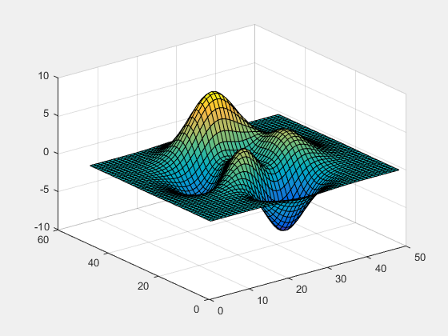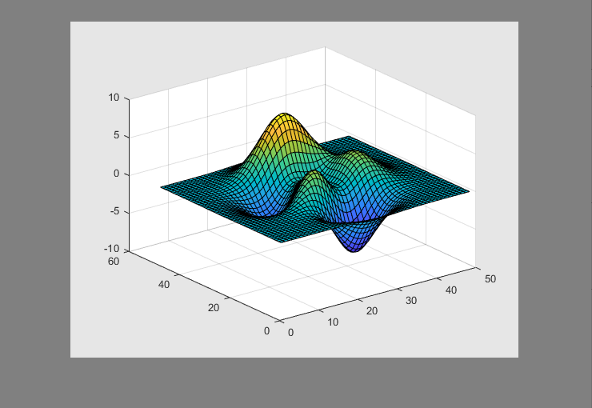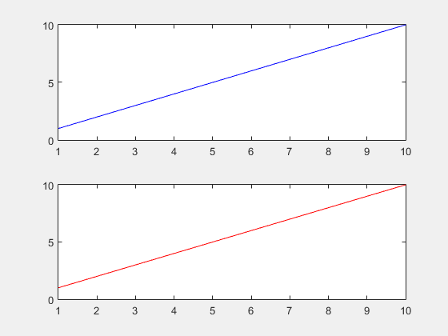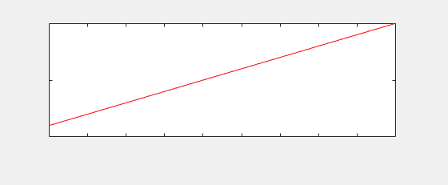# getframe

## 语法

``F = getframe``
``F = getframe(ax)``
``F = getframe(fig)``
``F = getframe(___,rect)``

## 说明

``F = getframe` 捕获显示在屏幕上的当前坐标区作为影片帧。`F` 是一个包含图像数据的结构体。`getframe` 按照屏幕上显示的大小捕获这些坐标区。它并不捕获坐标区轮廓外部的刻度标签或其他内容。`

``F = getframe(ax)` 捕获 `ax` 标识的坐标区而非当前坐标区。`

``F = getframe(fig)` 捕获由 `fig` 标识的图窗。如果您需要捕获图窗窗口的整个内部区域（包括坐标区标题、标签和刻度线），则指定一个图窗。捕获的影片帧不包括图窗菜单和工具栏。`
``F = getframe(___,rect)` 捕获 `rect` 定义的矩形内的区域。指定 `rect` 作为 `[left bottom width height]` 形式的四元素向量。将此选项用于上一语法中的 `ax` 或 `fig` 输入参数。 `

## 示例

```plot(rand(5)) F = getframe;````F` 是一个结构体，其中包含的 `cdata` 字段中包含捕获的图像数据。

```figure imshow(F.cdata)``````surf(peaks) F = getframe(gcf);````F` 是一个结构体，其中包含的 `cdata` 字段中包含捕获的图像数据。

```figure('Color',[0.5 0.5 0.5]) imshow(F.cdata)````plot(rand(5))````drawnow ax = gca; ax.Units = 'pixels'; pos = ax.Position```
```pos = 73.8000 47.2000 434.0000 342.3000```

```marg = 30; rect = [-marg, -marg, pos(3)+2*marg, pos(4)+2*marg]; F = getframe(gca,rect); ax.Units = 'normalized';```

```figure('Color',[0.5 0.5 0.5]) imshow(F.cdata)``````plot(rand(5)) xlabel('x values') title('Plot of Random Data')``````drawnow ax = gca; ax.Units = 'pixels'; pos = ax.Position; ti = ax.TightInset;```

```rect = [-ti(1), -ti(2), pos(3)+ti(1)+ti(3), pos(4)+ti(2)+ti(4)]; F = getframe(ax,rect);```

```figure('Color',[0.5 0.5 0.5]) imshow(F.cdata)``````tiledlayout(2,1) ax1 = nexttile; plot(1:10,'b') ax2 = nexttile; plot(1:10,'r')````F = getframe(ax2);`

```figure imshow(F.cdata)``````Z = peaks; surf(Z) axis tight manual ax = gca; ax.NextPlot = 'replaceChildren'; loops = 40; F(loops) = struct('cdata',[],'colormap',[]); for j = 1:loops X = sin(j*pi/10)*Z; surf(X,Z) drawnow F(j) = getframe(gcf); end ```

```fig = figure; movie(fig,F,2)```

## 输入参数

`getframe` 捕获包围坐标区轮廓的最小矩形中的内容。如果您要捕获所有刻度值和标签，则改用 `fig` 输入参数。

## 输出参数

• `cdata` - 存储为 `uint8` 值数组的图像数据。图像数据数组的大小取决于您的屏幕分辨率。

• `colormap` - 颜色图。在真彩色系统中，此字段为空。

• 如果您查询 `getframe` 捕获的区域（图窗、坐标区或由 `rect` 指定的区域）的大小，则以像素为单位的大小可能与 `cdata` 中的元素数不匹配。这种差异是因为 `cdata` 中的元素数取决于您的屏幕分辨率（以及操作系统设置），而 MATLAB® 中的像素数可能不对应于屏幕上的实际像素数。

• 从 R2015b 开始，如果您使用高分辨率系统，则 `cdata` 的大小可能大于在以前版本中或在其他系统上的大小。

## 局限性

• MATLAB Online™Web Apps (MATLAB Compiler) 中，`getframe` 不支持以下功能：

• 捕获使用 `uifigure` 函数创建的图窗的内容或图窗中的任何坐标区。

• 捕获使用 App 设计工具创建的 App 的内容或 App 中的任何坐标区。

## 详细信息

### 像素

• 在 Windows 系统上，一个像素是 1/96 英寸。

• Macintosh 系统上，一个像素是 1/72 英寸。

## 提示

• 为了在使用 `getframe` 值获得最快的性能，请确保图窗在屏幕上可见。如果图窗不可见，`getframe` 仍可捕获该图窗，但性能可能会减慢。

• 为了更好地控制图像数据的分辨率，请改用 `print` 函数。带有 print 的 `cdata` 输出参数返回图像数据。`resolution` 输入参数控制图像的分辨率。